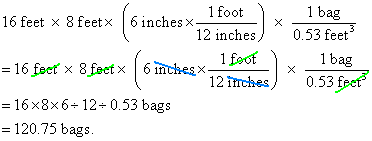SEARCH HOMEMath Central Quandaries & QueriesQuestion from Linda: I have a space of 16 feet by 8 feet. I want to fill it with pea gravel 6 inches deep. If the bags of pea gravel are .53 cubic foot. How many bags would I need. Thanks so much.Hi Linda.

When you multiply the length, width and depth, you get the volume. If you convert the depth to feet, then you get the volume in cubic feet. Then you can divide by the volume of gravel in each bag. Basically, you need to ensure that the units cancel out properly, leaving you with the right answer.Cheers,
Stephen La RocqueMath Central is supported by the University of Regina and The Pacific Institute for the Mathematical Sciences.